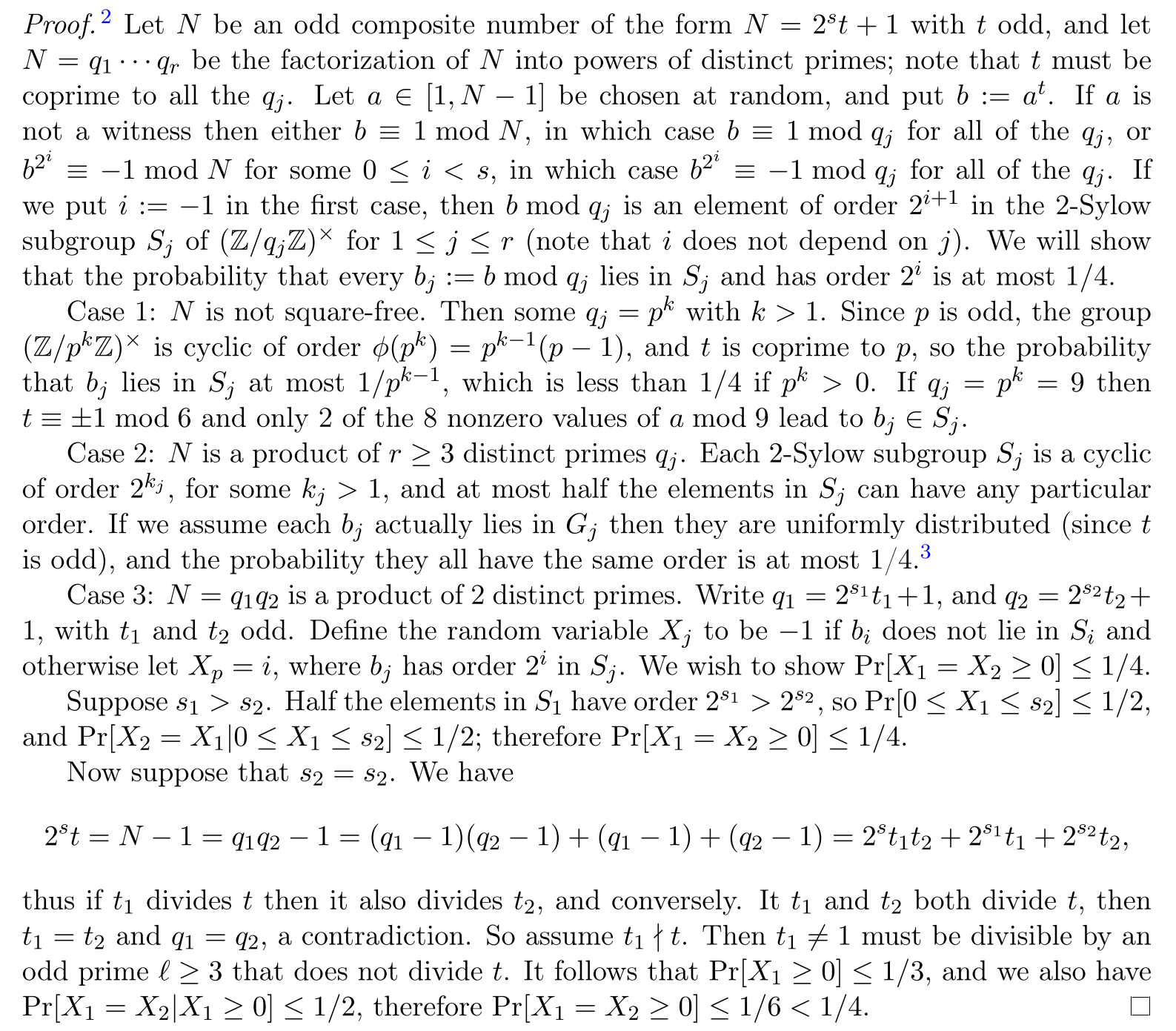## 卡米歇尔数#

• $n$是卡米歇尔数的充分必要条件是：
• $n$无平方因子
• $n$的每一个素因子$p$，有$p-1\mid n-1$
• $n$是奇数且至少有三个不同的素因子

## Miller Rabin Algorithm#

Miller Rabin Algorithm 的算法流程的核心就是，检验这些因子之中是否有数能被$p$整除。

Given an odd integer $N$:

1. Pick $a$ random integer $a\in[1,N-1]$.
2. Write $N = 2^st + 1$, with $t$ odd, and compute $b = a^t \mod N$. If $b ≡ \pm1 \pmod{N}$, return true ($a$ is not a witness, $N$ could be prime).
3. For $i$ from $1$ to $s − 1$: a. Set $b \leftarrow b^2$ mod $N$. b. If $b \equiv −1 \pmod{N}$, return true ($a$ is not a witness, $N$ could be prime).
4. Return false ($a$ is a witness, $N$ is definitely not prime).

（这里的witness是指数a是该数为合数的一个凭证

### 出错概率以及设置检验次数为多少比较合适#

Miller-Rabin 甚至可以筛掉一些卡米歇尔数。

（应该挺显然的，但我当时看了很久……呜呜)

$\textrm{Proof:}$

$$S=\set{a:a\in Z_n^*,(a,n)=1,[n\mid(a^k-1)]\lor[n\mid(a^{k2^i}+1)]}$$### 一个可以确保检验结果绝对正确的a集合#

（INT_64，Jim Sinclair）2

 1  2, 325, 9375, 28178, 450775, 9780504, 1795265022 

## Code#

• python3代码通过全部测试点用时6173ms
• cpp代码通过全部测试点用时447ms
• 随机数写法的python3的代码：
  1 2 3 4 5 6 7 8 9 10 11 12 13 14 15 16 17 18 19 20 21 22 23 24 25 26 27 28 29 30 31  import random import sys def miller_rabin(n : int) -> bool: if n <= 1: return False if n == 2: return True k, t = n - 1, 0 while not k & 1: k, t = k >> 1, t + 1 if t == 0: # n是大于2的偶数 return False for i in range(0, 10): a = random.randint(2, n - 1) tmp = pow(a, k, n) if tmp <= 1 or tmp == n - 1: continue for j in range(1, t + 1): if j == t: # 所有因子都不能被n整除 return False tmp = tmp * tmp % n if tmp == n - 1: break return True for num in sys.stdin: if miller_rabin(int(num)): print('Y') else: print('N') 
• 使用SPRP的python3代码：
  1 2 3 4 5 6 7 8 9 10 11 12 13 14 15 16 17 18 19 20 21  import sys def miller_rabin(n : int) -> bool: if n < 2 or n % 2 == 0: return n == 2 k, t = n - 1, 0 while ~k & 1: k, t = k >> 1, t + 1 for a in {2, 325, 9375, 28178, 450775, 9780504, 1795265022}: tmp = pow(a, k, n) if tmp <= 1 or tmp == n - 1: continue for j in range(0, t + 1): if j == t: return False tmp = pow(tmp, 2, n) if tmp == n - 1: break; return True for num in sys.stdin: if miller_rabin(int(num)): print('Y') else: print('N') 
• 但是python3的效率实在太慢，不能在算法竞赛中使用，下面给出c++的代码作为模板。
  1 2 3 4 5 6 7 8 9 10 11 12 13 14 15 16 17 18 19 20 21 22 23 24 25 26 27 28  using ull = unsigned long long; ull modmul(ull a, ull b, ull M) { ll ret = a * b - M * ull(1.L / M * a * b); return ret + M * (ret < 0) - M * (ret >= (ll)M); } ull modpow(ull b, ull e, ull mod) { ull ans = 1; for (; e; b = modmul(b, b, mod), e /= 2) if (e & 1) ans = modmul(ans, b, mod); return ans; } bool miller_rabin(ull n) { static const vector SPRP = { 2, 325, 9375, 28178, 450775, 9780504, 1795265022 }; if (n == 1 || n % 6 % 4 != 1) return (n | 1) == 3; ll t = __builtin_ctzll(n - 1), k = (n - 1) >> t; for (const ull &a : SPRP) { ull tmp = modpow(a, k, n); if (tmp <= 1 || tmp == n - 1) continue; for (int i = 0; i <= t; i++) { if (i == t) return false; tmp = modmul(tmp, tmp, n); if (tmp == n - 1) break; } } return true; }